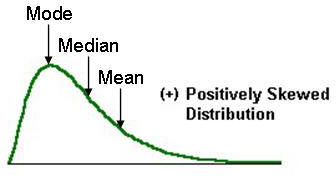2128 Measures Of Centre

8 Questions | Total Attempts: 678SettingsUse your knowledge of Mean, Median and Mode to answer the following questions.

• 1.
From the image above, which statement is most correct?
• A.

The mean and mode are the same

• B.

The median value is higher than the mean

• C.

The mean value is higher than the median

• D.

The mean and median are approximately the same

• 2.
From the image above, which statement is most correct?
• A.

The mean and mode are the same

• B.

The median value is higher than the mean

• C.

The mean value is higher than the median

• D.

The mean and median are approximately the same

• 3.
From the image above, which statement is most correct?
• A.

The mean and mode are the same

• B.

The median value is higher than the mean

• C.

The mean value is higher than the median

• D.

The mean and median are approximately the same

• 4.
From the image above, which statement is most correct?
• A.

The mean and mode are the same

• B.

The median value is higher than the mean

• C.

The mean value is higher than the median

• D.

The mean and median are approximately the same

• 5.
From the image above, which statement is most correct?
• A.

The mean and mode are the same

• B.

The median value is higher than the mean

• C.

The mean value is higher than the median

• D.

The mean and median are approximately the same

• 6.
From the image above, which statement is most correct?
• A.

The mean and mode are the same

• B.

The median value is higher than the mean

• C.

The mean value is higher than the median

• D.

The mean and median are approximately the same

• 7.
From the image above, which statement is most correct?
• A.

The mean and mode are the same

• B.

The median value is higher than the mean

• C.

The mean value is higher than the median

• D.

The mean and median are approximately the same

• 8.
From the image above, which statement is most correct?
• A.

The mean and mode are different

• B.

The median value is higher than the mean

• C.

The mean value is higher than the median

• D.

The mean and median are approximately the same

• 9.
From the image above, which statement is most correct?
• A.

The mean and mode are the same

• B.

The median value is higher than the mean

• C.

The mean value is higher than the median

• D.

The mean and median are approximately the same

• 10.
From the image above, which statement is most correct?
• A.

The mean and mode are the same

• B.

The median value is higher than the mean

• C.

The mean value is higher than the median

• D.

The mean and median are approximately the same

• 11.
From the image above, which statement is most correct?
• A.

The mean and mode are the same

• B.

The median value is higher than the mean

• C.

The mean value is higher than the median

• D.

The mean and median are approximately the same

Related TopicsBack to top